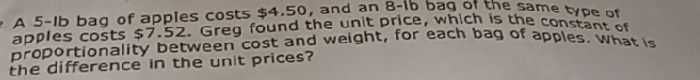### ¿Todavía tienes preguntas de matemáticas?

Pregunte a nuestros tutores expertos
Algebra
PreguntaA 5-Ib bag of apples costs $$\ 4.50 ,$$ and an $$8$$ -ib bag of the same type of apples costs $$\ 7.52$$ . Greg found the unit brice, which is the constant of proportionality between cost and weight, for each bag of apples. What is the difference in the unit prices?

$$y \approx 1.007 x - 0.533$$
$$y = \frac { 151 } { 150 } x - \frac { 8 } { 15 }$$